### 精度不变，两行代码无痛实现推理加速1.34倍，极市平台联合OpenVINO™加速AI开发部署openlab_4276841a 更新于 1月前

### 无痛实现 1.34 倍加速推理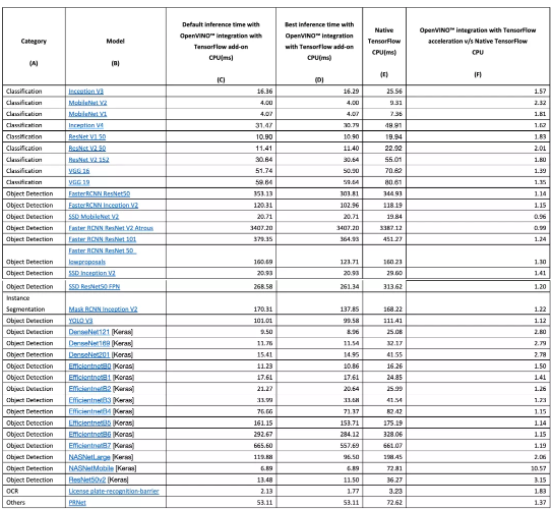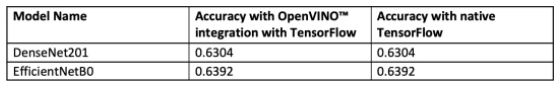### 具体实操

OpenVINO™ 与 TensorFlow 的集成专为使用 OpenVINO™ 工具套件的开发人员而设计——通过最少的代码修改来提高推理应用程序的性能。该集成为提高TensorFlow 兼容性提供以 OpenVINO™ 工具套件内联优化和所需运行时，并加速了各种英特尔芯片上多类AI模型的推理。

``import openvino_tensorflowopenvino_tensorflow.set_backend('<backend_name>')``

OpenVINO™ 与 TensorFlow 的集成通过将 TensorFlow 图巧妙地划分为多个子图，再将这些子图分派到 TensorFlow 运行时或 OpenVINO™ 运行时，从而实现最佳加速推理。

# 工作流概述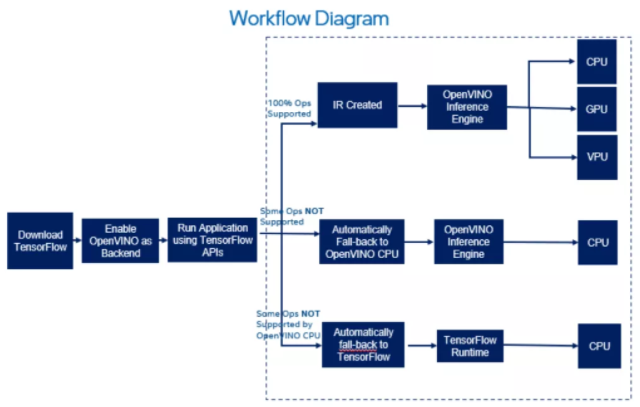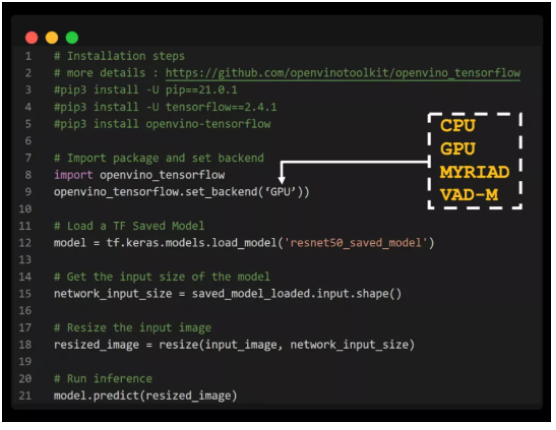https://github.com/openvinotoolkit/openvino_tensorflow

# 在Extreme Vision notebooks中集成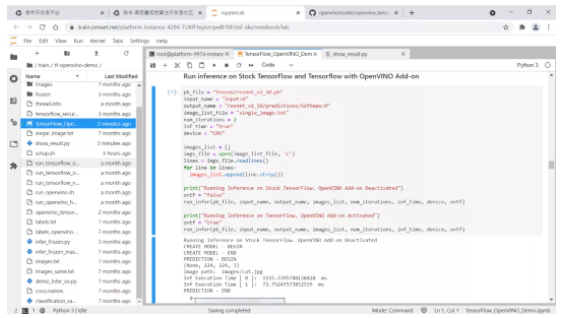### 对推理加速更深的讨论

#01 OPENVINO_TF_MIN_NONTRIVIAL_NODES 变量

#02 冻结 Keras 模型以获得最佳性能

``import tensorflow as tffrom tensorflow import kera***r>from tensorflow.keras.applications.densenet import DenseNet121# Add two lines of code to enable OpenVINO integration with TensorFlowimport openvino_tensorflowopenvino_tensorflow.set_backend("CPU")model = DenseNet121(weights='imagenet')# Run the inference using Keras API    model.predict(input_data)``

``import tensorflow as tffrom tensorflow import kera***r>from tensorflow.keras.applications.densenet import DenseNet121from tensorflow.python.framework.convert_to_constants import convert_variables_to_constants_v2# Add two lines of code to enable OpenVINO integration with TensorFlowimport openvino_tensorflowopenvino_tensorflow.set_backend("CPU")model = DenseNet121(weights='imagenet')# Freeze the model first to achieve the best performance# using OpenVINO integration with TensorFlow    full_model = tf.function(lambda x: self.model(x))full_model = full_model.get_concrete_function(tf.TensorSpec(model.inputs.shape,model.inputs.dtype, name=model.inputs.name))frozen_func = convert_variables_to_constants_v2(full_model)frozen_func.graph.as_graph_def()session = tf.compat.v1.Session(graph=frozen_func.graph)prob_tensor = frozen_func.graph.get_tensor_by_name(full_model.outputs.name)# Run the inference on the frozen modelsession.run(prob_tensor, feed_dict={full_model.inputs.name : input_data})``